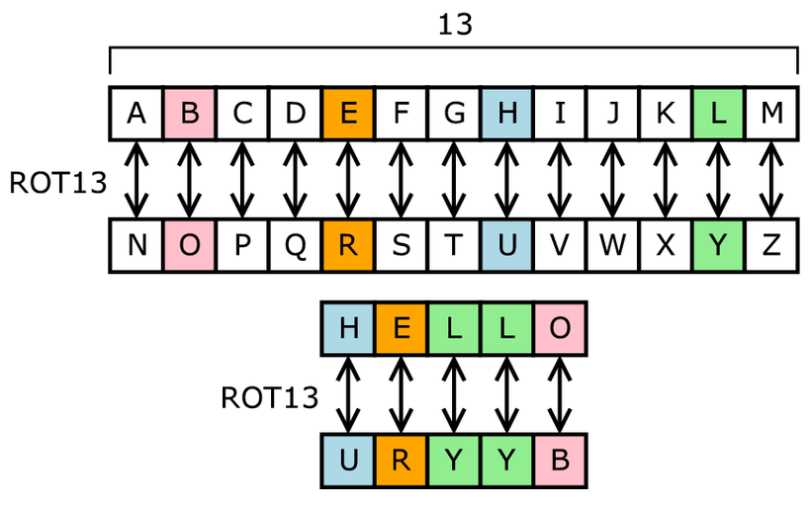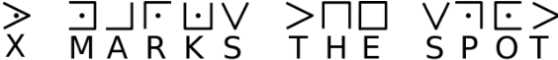# Implementing a Substitution Cipher in JavaScriptWritten by
Published on
Modified on
Category

Cipher's are a good way to put your coding skills into practice. They are usually relatively simple to implement in terms of the amount of code that you need, but they are complex in their logic.

And there's something cool about encrypting a message, sending it off, and having someone translate it on the other side.

*Note: Do not use a cipher in any professional setting. In the 1300's they might have been secure, but they are outdated by today's standards and can easily be cracked by brute force attempts.

## What is it?Before we start I will mention that there are various kinds of Substitution Ciphers that you can implement, ranging from very simple single letter-by-letter substitutions, as in the image above, to more complex multiple-letter grouping substitutions where a single letter (like 'a') can become multiple characters (like 'de').

The version shown above is one of the simplest examples because the alphabet that will be used to encode the original message, is simply a shifted version of the original alphabet (13 shifts in this case).

In more complex scenarios however, you might find that the encryption alphabet has no actual pattern and is mainly a randomly shuffled alphabet.

In this article, I will mainly be discussing the simpler case of a substitution cipher, however you can use this as a base for other more complicated sequences.

## Implementation

There are 2 approaches that you can take when designing the new alphabet that will be used as the substitution.

You can use the simpler shift alphabet approach, a version of a Ceaser Cipher, or you can use a mixed alphabet approach in which the new alphabet is randomly generated.

Let's check out both.

## Shifted alphabet

As mentioned, a shifted alphabet is essentially a Caesar Cipher. You essentially shift the alphabet by 'n' number of characters either left or right, and you wrap around once you get to the end.

A two letter shift to the right on the standard alphabet, would look like the following:

``````Normal text: ABCDEF...

Ciphertext: CDEFGH...``````

In which case, the bottom alphabet would become the substitution that we will use for the encoding.

Let's start by declaring a variable for the original alphabet.

``var alphabet = "abcdefghijklmnopqrstuvwxyz";``

The following function will be responsible for creating the new shifted alphabet.

``````function shift(n){
let newalpha = '';

for (let i = 0; i < alphabet.length; i++){
let offset = (i + n) % alphabet.length;
newalpha += alphabet[offset];
}

return newalpha;
}``````

This function essentially loops through each letter in the original alphabet and finds its new shifted sibling. If the shift value is greater than the length of the original alphabet, then the cursor will wrap around back to the beginning.

## Mixed alphabet

The shifted alphabet method above has one built in flaw that you can't really get away from. And that's the fact that you can only shift, at most, the length of the alphabet, meaning 26 possible rotations.

That wouldn't take even a standard laptop much time to crack these days.

The mixed alphabet approach however is exponentially more complex, as there is no real pattern there to figure it out.  A potential mixed alphabet could look something like this:

``````Original alphabet: ABCDEFG...

Mixed alphabet: HWAGQPOD...``````

You can generate a new mixed alphabet, by simply switching 2 random values in the original alphabet over and over again.

``````function shift(alphabet){
let newalpha = Array.from(alphabet);

for (let i = 0; i < 1000; i++)
{
let location1 = Math.floor((Math.random() * newalpha.length));
let location2 = Math.floor((Math.random() * newalpha.length));
let tmp = newalpha[location1];

newalpha[location1] = newalpha[location2];
newalpha[location2] = tmp;
}

return newalpha.join('');
}``````

The best part here is that the encoding and decoding functions don't really care what the secondary alphabet looks like. It will use whichever it is given in order to perform the substitution.

Once you have your new lookup alphabet generated, it's time to perform the actual substitution.

## Substitution

Single letter substitutions are the simplest to perform as they just require a simple 1 to 1 look up from one alphabet to another.

Here is a simple implementation of that function:

``````var alphabet = "abcdefghijklmnopqrstuvwxyz";

function encode(message){
let result = "";
let newalpha = shift(alphabet);

message = message.toLowerCase();
for (let i = 0; i < message.length; i++){
let index = alphabet.indexOf(message[i]);
result += newalpha[index];
}
return result;
}``````

Multiple letter substitutions however can be much more complex in nature, depending on how you plan on setting them up.

In that scenarios, a given letter (such as 'a') would be substituted to a multiple character string.

``````Original alphabet: abcdefg...
New alphabet: de ee ui ew qf...``````

In this case, each single letter would translate as a sequence of 2 letters, making the translation more difficult to make out, those at twice the cost in terms of size.

Other methods of substitution involve using symbols, instead of letters in order to encode the given message. The pigpen cipher is one example of this:The more complex the substitution though, the more difficult it is to implement it. Note that whether you use letters or symbols to do the substitution there is no inherent increase in security, as there is still a 1 to 1 correlation.

## Grouping

To make things more difficult to read, often times you will see the encoded result of a message split into groups of letters, such as 4 or 5.

``SIAAZ QLKBA VAZOA RFPBL UAOAR``

This makes determining where words begin and end much more difficult overall, as often times spaces can be used to figure out word length and frequency.

With this approach though, you might lose the ability to decode the message back to its original state (using spaces as word breaks), unless you specifically encode the spaces as well.

## Security

Generating random alphabets is overall better than working with shifted alphabets. As mentioned above, you can only shift an alphabet 26 times given the standard English letter set.

Generating random permutations though can yield up to 288.4 possible results. That's a much higher complexity rate over a standard shifted substitution though note that it is still not a very strong cipher as a whole.

By analyzing the frequency distribution of certain characters, a cryptoanalyst can easily brute force their way into the decoded message, even with a complex substitution pattern.

But overall, substitution ciphers are a fun way to practice your overall algorithmic coding skills.Walter Guevara is a software engineer, startup founder and currently teaches programming for a coding bootcamp. He is currently building things that don't yet exist.